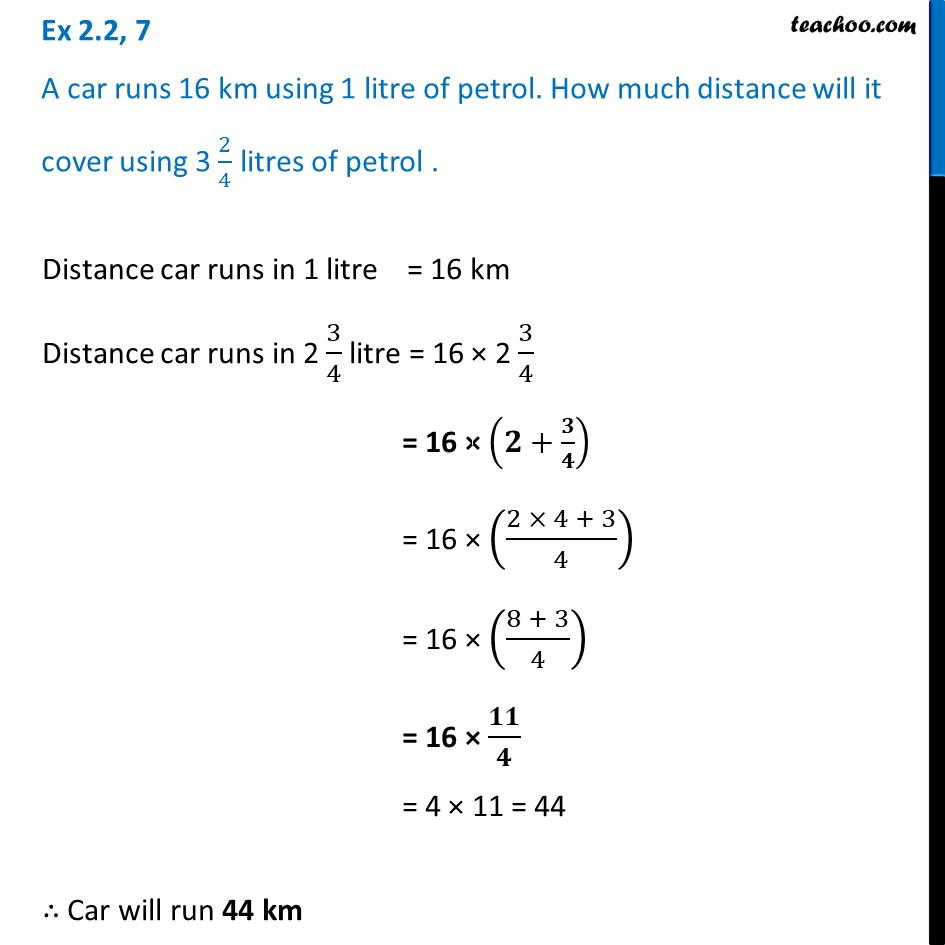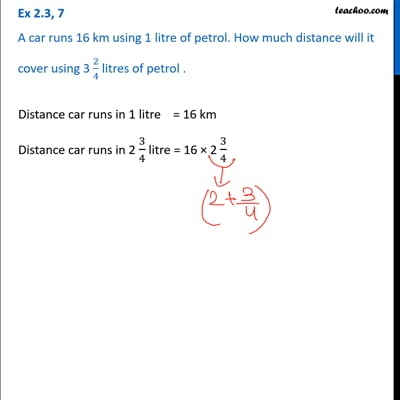Multiplication of Fractions - Statement Questions

Chapter 2 Class 7 Fractions and Decimals
Concept wiseThis video is only available for Teachoo black users

Learn in your speed, with individual attention - Teachoo Maths 1-on-1 Class

### Transcript

Ex 2.2, 7 A car runs 16 km using 1 litre of petrol. How much distance will it cover using 3 2/4 litres of petrol . Distance car runs in 1 litre = 16 km Distance car runs in 2 3/4 litre = 16 × 2 3/4 = 16 × (𝟐+𝟑/𝟒) = 16 × ((2 × 4 + 3)/4) = 16 × ((8 + 3)/4) = 16 × 𝟏𝟏/𝟒 = 4 × 11 = 44 ∴ Car will run 44 km